• 手动爬取的知乎问题数据，共十万条，已做过简单清洗数据集格式描述如下： id | 标题 | 回答数 | 关注数 | 浏览数 | 标签 【仅供学习使用，请勿商用】
• 《Python3爬虫、数据清洗与可视化》pdf+配套代码+数据集，亲测全部有效，提供给大家学习交流！欢迎下载python 爬虫
• Python3爬虫、数据清洗与可视化配套资源代码和数据集 零一 韩要宾 黄园园 Python技术入门读物，通过实战教初学者
• 数据收集：网络爬虫、公开数据集、客户数据 数据处理：数据清洗、数据规整 数据分析：数据统计、探索性数据分析(EDA)、数据建模 结果展示：数据可视化、报表生成、结果保存 数据收集：获取歌单索引页、...
• 中科院李子青课题组于2014年发布的CASIA-WebFace数据集收集了10000多个名人的照片，然而，这些照片是在网上用爬虫扒下来的，所以有一些数据存在问题，这里对这个数据集清洗。 1：使用RetinaFace对给定的图片做人脸...
中科院李子青课题组于2014年发布的CASIA-WebFace数据集收集了10000多个名人的照片，然而，这些照片是在网上用爬虫扒下来的，所以有一些数据存在问题，这里对这个数据集做清洗。 1：使用RetinaFace对给定的图片做人脸检测，对于提取不到landmark/boundingbox的图片逐个做分析。 2：将图像缩小送进网络训练发现很多都可以检测出来了，其实retinaface对尺度比较小的图片效果会好很多
展开全文CASIA数据集
• 爬取完数据之后，需要对数据进行清洗，使其成为能够被我们利用的数据集。这里我爬取了一个手机尺寸的网站，准备计算得到其高宽信息，并生成了一个数据集。后面会根据这个数据集进行一下分类操作。 当然现在如果把...
前言
机器学习很重要的一部分就是数据，因此可以通过爬虫爬取一些数据。爬取完数据之后，需要对数据进行清洗，使其成为能够被我们利用的数据集。这里我爬取了一个手机尺寸的网站，准备计算得到其高宽信息，并生成了一个数据集。后面会根据这个数据集进行一下分类操作。 当然现在如果把爬虫爬到的数据用于商业，现在很多时候是不合法的，还是要注意点。
准备工作
这里使用的是python 3.6进行实现的，需要安装如下一些库：
beautifulsoup4
requests
lxml
tensorflow
scikit-learn
pandas
matplotlib

这些库一部分是后面四个是用于分类的，可以先不下。我们这边就使用了requests+beautifulsoup+lxml爬取数据并进行结构分析。
主要工作
发送请求下载页面
可以直接解析页面，但保险起见还是先下载下来然后在进行处理比较好，不然重复请求别人的网页也不是很好也没什么必要，所以写了一个从txt中读取保存页面的函数。 因为有好几个页面，所以循环调用了好次，将页面都保存为txt文件。主要函数如下：
PAGES = ['index', 'pad', 'watch', 'computer', 'display']

def get_page_content_crawler(url, page_name):
try:
'User-Agent': 'Mozilla/5.0 (Windows NT 10.0; WOW64) AppleWebKit/537.36 (KHTML, like Gecko) Chrome/70.0.3538.67 Safari/537.36',
}
url = url % page_name
page_content.encoding = 'utf-8'
content = page_content.text
save_page_content2txt(content, page_name)
return content
except:
s = sys.exc_info()
print("Error '%s' happened on line %d" % (s, s.tb_lineno))
return "ERROR"

def get_page_content_from_txt(page_name):
with open('%s.txt' % page_name, 'r') as f:
soup = BeautifulSoup(html, 'lxml')  # 采用lxml进行解析
return soup

def save_page_content2txt(content, page_name):
with open('%s.txt' % page_name, 'w') as f:
for i in content:
f.write(i)

读取页面并解析
页面如下所示，是一个表格：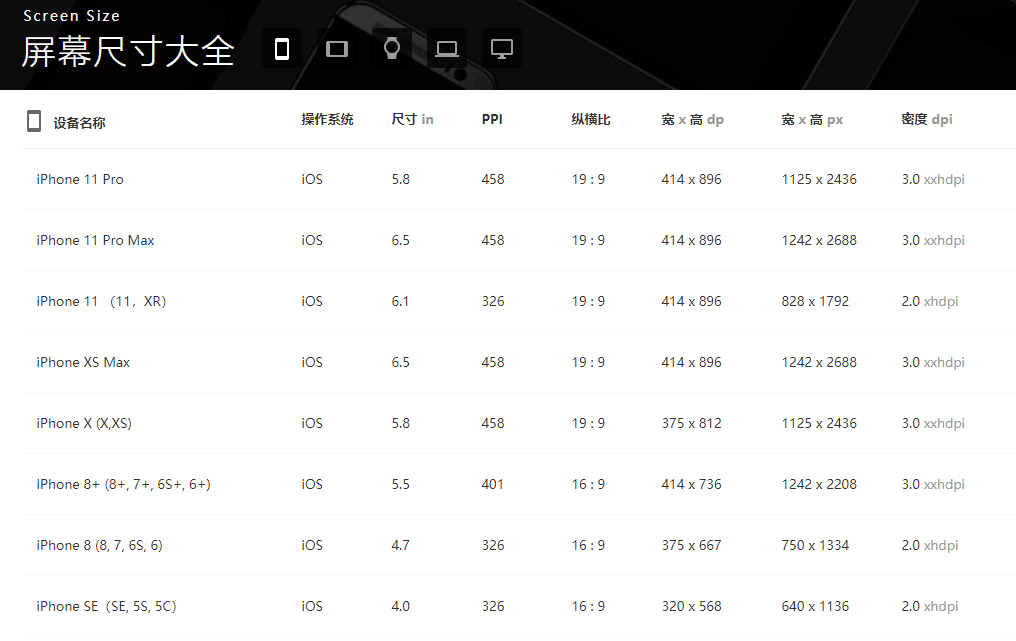分析网页html的结构如下，可以发现有且仅有一个tbody，而每个设备的信息都是存在一个tr里面的，相关信息也是有对应的class。
<tbody>
<tr>
<td class="td01" valign="middle">iPhone 11 Pro</td>
<td class="td02" valign="middle">iOS</td>
<td class="td03" valign="middle">5.8</td>
<td class="td04" valign="middle">458</td>
<td class="td05" valign="middle">19 : 9</td>
<td class="td06" valign="middle">414 x 896</td>
<td class="td07" valign="middle">1125 x 2436</td>
<td class="td08" valign="middle">3.0
<font class="gray01">xxhdpi</font>
</td>
</tr>
......

因此撰写结构分析的代码：
def extract_content2excel(soup, page_name):
all_devices = soup.find('tbody')
one_device = all_devices.find_all_next('tr')
with open('%s.csv' % page_name, 'w') as f:
writer = csv.writer(f)
header = ['name', 'device_os', 'size', 'ppi', 'aspect_ratio', 'wh_dp', 'wh_px', 'dpi']
for device in one_device:
name = device.find('td', class_='td01').text
device_os = device.find('td', class_='td02').text.replace(' ', '')
size = device.find('td', class_='td03').text.replace(' ', '')
ppi = device.find('td', class_='td04').text.replace(' ', '')
aspect_ratio = device.find('td', class_='td05').text.replace(' ', '')
wh_dp = device.find('td', class_='td06').text.replace(' ', '')
wh_px = device.find('td', class_='td07').text.replace(' ', '')
dpi = device.find('td', class_='td08').text.replace(' ', '').replace('\n', '')
content = [name, device_os, size, ppi, aspect_ratio, wh_dp, wh_px, dpi]
writer.writerow(content)

将其中一些空格去除，然后存入一个csv文件中，多个页面会有多个文件。
生成数据集
整合多个csv文件
csv文件是以逗号为分割符的，有多种方式可以读取。爬取的数据如下，我们可以知道手机屏幕是多少吋，一般是指对角线的长度，而1寸=2.54厘米，因此可以根据尺寸和纵横比计算手机的高和宽。
name,device_os,size,ppi,aspect_ratio,wh_dp,wh_px,dpi
iPhone 11 Pro,iOS,5.8,458,19:9,414x896,1125x2436,3.0xxhdpi
iPhone 11 Pro Max,iOS,6.5,458,19:9,414x896,1242x2688,3.0xxhdpi
iPhone 11 （11，XR）,iOS,6.1,326,19:9,414x896,828x1792,2.0xhdpi
iPhone XS Max,iOS,6.5,458,19:9,414x896,1242x2688,3.0xxhdpi
.......

这里取第三列和第五列的数据即可，根据上面的公式计算得到高和宽：
def read_csv(page_name):
'''
读取csv中的数据
:param page_name:文件的名称
:return: np格式的str list(name, height, width)
'''
with open('%s.csv' % page_name, 'r', encoding='UTF-8') as f:
device_info = np.empty([0, 3])
for r in f_csv:
if r == '-' or r == '-':  # 清洗数据
continue
height, width = get_wh_from_size(r, r)
one_device = (r, height, width)  # 这里会导致height和width转化成str
device_info = np.row_stack((device_info, one_device))
return device_info

def get_wh_from_size(size, aspect_ratio):
'''
解析csv文件中的数据
:param size: 手机尺寸（吋表示对角线）
:param aspect_ratio: 纵横比
:return: 高、宽(单位已经转化为cm)
'''
ratio = map(float, aspect_ratio.split(':'))
height_ratio, width_ratio = list(ratio)
hypotenuse_ratio = math.sqrt(height_ratio**2 + width_ratio**2)
hypotenuse_cm = float(size) * 2.54
height = round((height_ratio * hypotenuse_cm / hypotenuse_ratio), 2)
width = round((width_ratio * hypotenuse_cm / hypotenuse_ratio), 2)
return height, width

可视化并保存数据集
使用添加的方式将每一行保存到data.csv中，这里写的不算特别好，因为每次添加一行都进行了一次文件打开的操作，有点消耗内存，可以考虑所有的存储为array再打开文件。
def draw_scatter():
# 拼接三个数据
devices = []
for page in PAGE:
devices.append(device_info)

all_data = []
for device in devices:
point = (device.T, device.T)
all_data.append(point)

colors = ('red', 'blue', 'green')

# Create plot
fig = plt.figure()

for data, color, group in zip(all_data, colors, groups):
x, y = data
print(group, "的数量是：", len(x))

index = groups.index(group)
save_data_csv(index, list(x.T), list(y.T))
print(x.shape, y.shape)
x = list(map(np.float32, x))
y = list(map(np.float32, y))

ax.scatter(x, y, alpha=1, c=color, edgecolors='none', s=30, label=group)
ax.axis('auto')
plt.title('Device Size Scatter')
plt.legend(loc=2)
plt.xlabel('height')
plt.ylabel('width')
plt.show()

def save_data_csv(label, *paras):
# 添加，newline使得新行无回车
with open('data.csv', 'a+', newline='') as f:
writer = csv.writer(f)
if label == 0:
writer.writerow(['height', 'width', 'label'])

paras = np.array(paras).T

for item in paras:
content = []
for para in item:
content.append(para)
content.append(label)
writer.writerow(content)

最终的数据分布如下：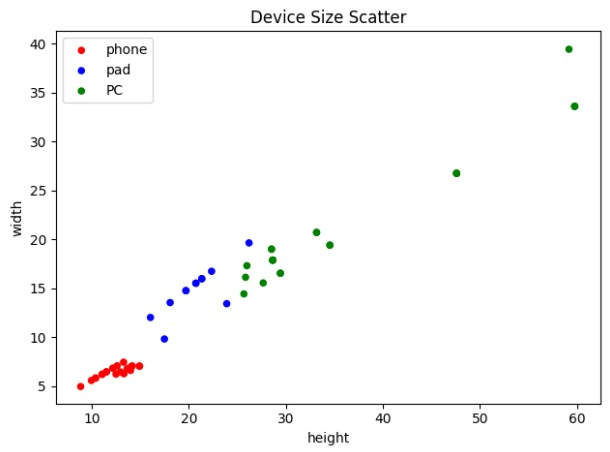数据格式如下，分为三列进行了保存：
height,width,label
13.31,6.31,0
14.92,7.07,0
14.0,6.63,0
14.92,7.07,0
...
20.73,15.54,1
16.05,12.04,1
21.34,16.0,1
...
25.85,16.15,2
25.68,14.45,2
28.65,17.9,2

完成数据集制作之后我们就可以随意进行操作了。
参考链接
https://www.jianshu.com/p/47908cd4f424https://uiiiuiii.com/screen
展开全文• 数据清洗 什么是数据清洗 1.数据清洗(Data cleaning)– 对数据进行重新审查和校验的过程，目的在于删除重复信息、纠正存在的错误，并提供数据一致性。 数据清洗从名字上也看的出就是把“脏”的“洗掉”，指发现并...
数据清洗
什么是数据清洗
1.数据清洗(Data cleaning)– 对数据进行重新审查和校验的过程，目的在于删除重复信息、纠正存在的错误，并提供数据一致性。
数据清洗从名字上也看的出就是把“脏”的“洗掉”，指发现并纠正数据文件中可识别的错误的最后一道程序，包括检查数据一致性，处理无效值和缺失值等。
为什么进行数据清洗
因为数据仓库中的数据是面向某一主题的数据的集合，这些数据从多个业务系统中抽取而来而且包含历史数据，这样就避免不了有的数据是错误数据、有的数据相互之间有冲突，

这些错误的或有冲突的数据显然是我们不想要的，称为“脏数据”
我们要按照一定的规则把“脏数据”“洗掉”，这就是数据清洗。

而数据清洗的任务是过滤那些不符合要求的数据，将过滤的结果交给业务主管部门，确认是否过滤掉还是由业务单位修正之后再进行抽取。不符合要求的数据主要是有不完整的数据、错误的数据、重复的数据三大类。

数据清洗是与问卷审核不同，录入后的数据清理一般是由计算机而不是人工完成

2.数据清洗的步骤
数据清洗的步骤相对较为简单与清晰，有两个阶段：
第一步：偏差检测（Discrepancy Detection）：即检查导致偏差的因素，并识别离散值与噪声值 例如我们采取的数据是否具有代表性，数据量是否足够，偶然因素，其他因素等的影响，这些都是能够体现数学模型上的离散值和噪声值
第二步：数据清洗（Data Cleansing）：即处理缺失值与噪声。
3.数据清洗的方法 缺失数据填补 填补缺失值的方法有如下3大类：
直接删除： 可以删除带有缺失值的记录，也可直接删除有缺失值的属性。但在实际中不太有效，尤其是在缺失值变化多，跨多个自变量的情况下。
人工填写： 用人手工填写上确实的值，听上去就不实际且费时间。大数据的分析可以直接忽略这个方法。
自动填充： 这个方法是目前用的最为广泛的。顾名思义，用某些值自动填充缺失值。依照自动填充的值不同做出清洗
4.那么我们该怎么实现数据清洗呢？
备注：其实不需要我们干，因为这些往往都是有专门的岗位或者部门进行的，例如算法工程师出算法，大数据工程师进行实现，运用大数据的相关框架/知识进行清洗。
ps:那么我们该怎么实现数据清洗呢，我们的做法是对爬虫获取到的数据实现统计求和或者去重排序等
java 利用爬虫清洗的步骤

import java.io.File;
import java.io.FileOutputStream;
import java.io.IOException;
import org.apache.poi.hssf.usermodel.HSSFCell;
import org.apache.poi.hssf.usermodel.HSSFRow;
import org.apache.poi.hssf.usermodel.HSSFSheet;
import org.apache.poi.hssf.usermodel.HSSFWorkbook;

/**
* @desc   poi实现数据写入excel中
* @author WYH
* @time   2019-10-13
*/
public class Test {

public static void main(String[] args) throws IOException {

//1.poi核心类的实例化
HSSFWorkbook workBook=new HSSFWorkbook();

//2.创建sheet
HSSFSheet sheet = workBook.createSheet();

//3.创建表头
HSSFRow row = sheet.createRow(0);
String []biaoTou={"编号","名字"};
for (int i = 0; i
{
HSSFCell cell = row.createCell(i);
cell.setCellValue(biaoTou[i]);
}

//3.填充20行数据
for(int i =1;i
{
HSSFRow row2 = sheet.createRow(i);//从第二行开始创建新的一行
HSSFCell cell2=row2.createCell(0);//为新创建的行创建第一列
cell2.setCellValue(i);	//给新创建的第一列赋值

HSSFCell cell3 = row2.createCell(1);
cell3.setCellValue("00-"+i);
}

File file =new File("F:/20191013.xls");
FileOutputStream outputStream = new FileOutputStream(file);
workBook.write(outputStream);
outputStream.flush();
outputStream.close();
workBook.close();
System.out.println("使用POI写入数据成功了.....");
}

}

import java.io.IOException;
import java.util.ArrayList;
import java.util.List;

import org.apache.poi.ss.usermodel.Workbook;

/**
* @desc   数据的清洗
* @author WYH
* @time   2019-10-13
*/
public class Test {

public static void main(String[] args) throws IOException {
List> list=new ArrayList>();
for (int i = 1; i
{
String pathname="D:/zp/excel/zhyc-java0-"+i+".xls";
ExcelUtils  excelUtils=new ExcelUtils();
Workbook workBook = excelUtils.getWorkBook(pathname);
// String result = excelUtils.getCell(workBook,int sheetNum,int beginColumn,int endColumn);
List resultList = excelUtils.getCell(workBook,0,2,2);
System.out.println(resultList);
}

int h=0,m=0,w=0;
for(int i =0;i
{
List everyResultList = list.get(i);
for(int k =0;k
{
String everyResult = everyResultList.get(k);
if (!(everyResult.startsWith("其他")||everyResult.startsWith("面议")||everyResult.startsWith("薪")))
{
int st = everyResult.lastIndexOf("-");//注意这个-建议要粘贴excel中的
String ft = (String) everyResult.subSequence(0, st);
//System.out.println(ft);
if (Integer.parseInt(ft)>=12000)
{
h++;
}
else if ((Integer.parseInt(ft)>=8000)&&(Integer.parseInt(ft)
{
m++;
}
else
{
w++;
}

}
}

}

System.out.println("12000元以上的有："+h+"人");
System.out.println("8000-12000元之间的有："+m+"人");
System.out.println("8000元以下的有："+w+"人");

}

}


数据可视化的操作
1.数据可视化 是关于数据视觉表现形式的科学技术研究。其中

这种数据的视觉表现形式被定义为，一种以某种概要形式抽提出来的信息，包括相应信息单位的各种属性和变量。

它是一个处于不断演变之中的概念，其边界在不断地扩大。主要指的是技术上较为高级的技术方法，而这些技术方法允许利用图形、图像处理、计算机视觉以及用户界面，通过表达、建模以及对立体、表面、属性以及动画的显示，对数据加以可视化解释。与立体建模之类的特殊技术方法相比，数据可视化所涵盖的技术方法要广泛得多
2.数据可视化的分类
1）广义上，可视化无处不在。网站是数据可视化, 背后是数据库密密麻麻的数据表, 到了浏览器就是浅显易懂的页面；淘宝是商品的可视化, 上面有价格、发货地种种过滤器；微信是实时数据的可视化, 围起了你的社交网络, 让你一眼看到最新的消息流。
2）狭义上的数据可视化, 更多是纯图形去代表数据
poi写入数据
package com.rj.bd.pois.poi01;

import java.io.File;
import java.io.FileOutputStream;
import java.io.IOException;
import org.apache.poi.hssf.usermodel.HSSFCell;
import org.apache.poi.hssf.usermodel.HSSFRow;
import org.apache.poi.hssf.usermodel.HSSFSheet;
import org.apache.poi.hssf.usermodel.HSSFWorkbook;

/**
* @desc   poi实现数据写入excel中
* @author WYH
* @time   2019-10-13
*/
public class Test {

public static void main(String[] args) throws IOException {

//1.poi核心类的实例化
HSSFWorkbook workBook=new HSSFWorkbook();

//2.创建sheet
HSSFSheet sheet = workBook.createSheet();

//3.创建表头
HSSFRow row = sheet.createRow(0);
String []biaoTou={"编号","名字"};
for (int i = 0; i
{
HSSFCell cell = row.createCell(i);
cell.setCellValue(biaoTou[i]);
}

//3.填充20行数据
for(int i =1;i
{
HSSFRow row2 = sheet.createRow(i);//从第二行开始创建新的一行
HSSFCell cell2=row2.createCell(0);//为新创建的行创建第一列
cell2.setCellValue(i);	//给新创建的第一列赋值

HSSFCell cell3 = row2.createCell(1);
cell3.setCellValue("00-"+i);
}

File file =new File("F:/20191013.xls");
FileOutputStream outputStream = new FileOutputStream(file);
workBook.write(outputStream);
outputStream.flush();
outputStream.close();
workBook.close();
System.out.println("使用POI写入数据成功了.....");
}

}

poi读取数据
package com.rj.bd.pois.poi01;

import java.io.FileInputStream;
import java.io.IOException;
import org.apache.poi.hssf.usermodel.HSSFRow;
import org.apache.poi.hssf.usermodel.HSSFSheet;
import org.apache.poi.hssf.usermodel.HSSFWorkbook;
import org.apache.poi.ss.usermodel.Cell;

/**
* @desc   利用POI实现读取excel中的数据
* @author WYH
* @time   2019-10-13
*/
public class Test02 {

public static void main(String[] args) throws IOException {

String pathname="F:/20191013.xls";
//将需要解析的excel转为流进行输出
FileInputStream  fileInputStream=new FileInputStream(pathname);
//实例化POI的核心类
HSSFWorkbook  workBook=new HSSFWorkbook(fileInputStream);
//获取当前工作簿中的第一个sheet也就是第一页
HSSFSheet sheet = workBook.getSheetAt(0);
//获取当前sheet中有多少个行
int totalRowNums = sheet.getPhysicalNumberOfRows();

for(int i =0;i
{
HSSFRow row = sheet.getRow(i);//取出每一行
int totalCellNums = row.getPhysicalNumberOfCells();//获取一共有多少列
for(int k=0;k
{
Cell cell = row.getCell(k);
//System.out.print(cell.getStringCellValue());
String cellValue = getValue(cell);
System.out.print(cellValue+"\t");

}
System.out.println();
}

}

/**
* @desc  格式化输出
* @param cell
* @return
*/
private static String getValue(Cell cell) {
if (cell.getCellType()==Cell.CELL_TYPE_NUMERIC)
{
int d = (int)cell.getNumericCellValue();
return String.valueOf(d);
}
else if (cell.getCellType()==Cell.CELL_TYPE_BOOLEAN)
{
String.valueOf(cell.getBooleanCellValue());
}
return cell.getStringCellValue();
}

}


展开全文• 一：数据挖掘 我选用了链家网做数据爬取场所（不得不唠叨一句，这个网站真是为了爬虫而生的，对爬虫特别友好哈哈哈，反扒措施比较少） 比如我们爬取贵阳市乌当区的所有房子的房价及其他信息： 比如我们爬取第一...
一：数据挖掘
我选用了链家网做数据爬取场所（不得不唠叨一句，这个网站真是为了爬虫而生的，对爬虫特别友好哈哈哈，反扒措施比较少）
比如我们爬取贵阳市乌当区的所有房子的房价及其他信息：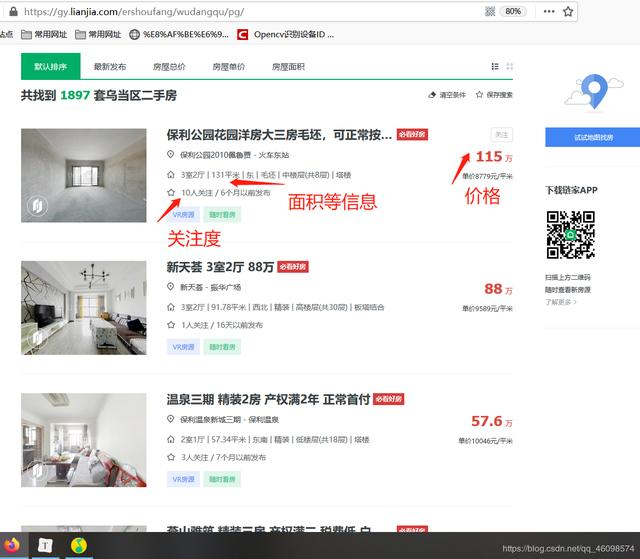比如我们爬取第一个房子的价格：115万：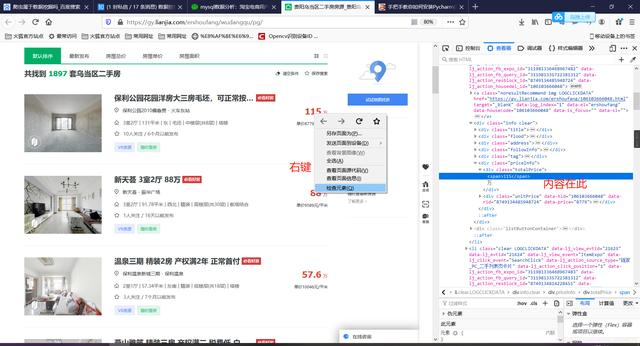接下来我们可以使用复制CSS选择器或者XPath等等来实现获取：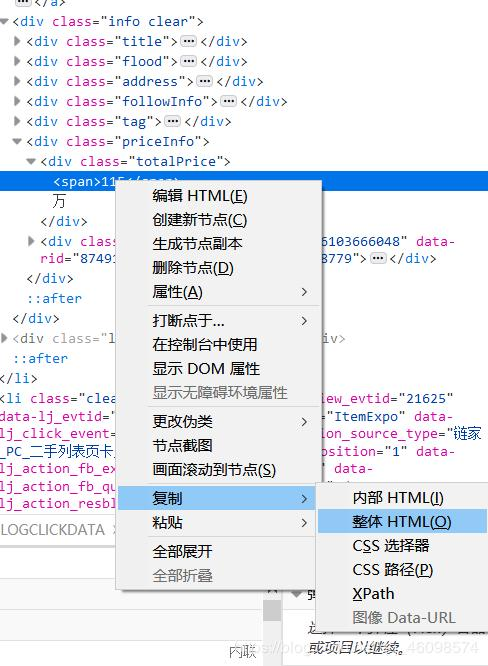下面我们使用复制XPath的方式，修改路径即可（需要一定前端知识）：
分别实现详解：
1:导入必备库
import requests
from lxml import etree
import xlwt
from xlutils.copy import copy
import xlrd
import csv
import pandas as pd
import time
细说一下：Requests 是用Python语言编写，基于 urllib，采用 Apache2 Licensed 开源协议的 HTTP 库，爬虫必备技能之一。它比 urllib 更加方便，可以节约我们大量的工作，完全满足 HTTP 测试需求。Requests 的哲学是以 PEP 20 的习语为中心开发的，所以它比 urllib 更加 Pythoner。更重要的一点是它支持 Python3 哦！
Pandas是python第三方库，提供高性能易用数据类型和分析工具 ， pandas是一个强大的分析结构化数据的工具集；它的使用基础是Numpy（提供高性能的矩阵运算）；用于数据挖掘和数据分析，同时也提供数据清洗功能。
2：定义爬取URL地址和设置请求头（其实还可以更完善，不过链家网比较友善，这点够用了）
self.url = 'https://gy.lianjia.com/ershoufang/wudangqu/pg{}/'
"User-Agent": "Mozilla/5.0 (Windows NT 10.0; WOW64) AppleWebKit/537.36 (KHTML, like Gecko) Chrome/75.0.3770.100 Safari/537.36"}

url是要获取信息的地址：我们选用贵阳市（gy）乌当区（wudangqu）为目标，然后pg{}是页码的意思：pg100就是爬第一百页，这里我们使用{}做一下占位，方便后续从第一页迭代到最后。
3：使用Requests获取数据
def get_response_spider(self, url_str):  # 发送请求
time.sleep(4)
response = get_response.content.decode()
html = etree.HTML(response)
return html
4：使用Xpath筛选数据源,过程见上图，需要一定的前端知识，不过，也有一些技巧：
def get_content_html(self, html):  # 使xpath获取数据
self.houseInfo = html.xpath('//div[@class="houseInfo"]/text()')
self.title = html.xpath('//div[@class="title"]/a/text()')
self.positionInfo = html.xpath('//div[@class="positionInfo"]/a/text()')
self.totalPrice = html.xpath('//div[@class="totalPrice"]/span/text()')
self.unitPrice = html.xpath('//div[@class="unitPrice"]/span/text()')
self.followInfo = html.xpath('//div[@class="followInfo"]/text()')
self.tag = html.xpath('//div[@class="tag"]/span/text()')
5：使用生成器，通过for循环和yield生成器迭代生成数据项：
def xpath_title(self):
for i in range(len(self.title)):
yield self.title[i]

def xpath_positionInfo(self):
for i in range(len(self.positionInfo)):
yield self.positionInfo[i]

def xpath_totalPrice(self):
for i in range(len(self.totalPrice)):
yield self.totalPrice[i]
def xpath_unitPrice(self):
for i in range(len(self.unitPrice)):
yield self.unitPrice[i]
def xpath_followInfo(self):
for i in range(len(self.followInfo)):
yield self.followInfo[i]
def xpath_tag(self):
for i in range(len(self.tag)):
yield self.tag[i]
6：通过调用这些函数进行预获得：
self.xpath_houseInfo()
self.xpath_title()
self.xpath_positionInfo()
self.xpath_totalPrice()
self.xpath_unitPrice()
self.xpath_followInfo()
self.xpath_tag()
get_houseInfo = self.xpath_houseInfo()
get_title = self.xpath_title()
get_positionInfo=self.xpath_positionInfo()
get_totalPrice = self.xpath_totalPrice()
get_unitPrice = self.xpath_unitPrice()
get_followInfo=self.xpath_followInfo()
get_tag=self.xpath_tag()
这里的函数就是调用上面的生成器的函数：生成器yield 理解的关键在于：下次迭代时，代码从yield的下一跳语句开始执行。
7：数据筛选，写入文本中：
while True:
data_houseInfo= next(get_houseInfo)
data_title=next(get_title)
data_positionInfo=next(get_positionInfo)
data_totalPrice=next(get_totalPrice)
data_unitPrice=next(get_unitPrice)
data_followInfo=next(get_followInfo)
data_tag=next(get_tag)

with open("lianjia1.csv", "a", newline="", encoding="utf-8-sig") as f:
fieldnames = ['houseInfo', 'title', 'positionInfo', 'totalPrice/万元', 'unitPrice', 'followInfo', 'tag']
writer = csv.DictWriter(f, fieldnames=fieldnames)  # 写入表头
list_1 = ['houseInfo', 'title', 'positionInfo', 'totalPrice/万元', 'unitPrice', 'followInfo', 'tag']
list_2 = [data_houseInfo,data_title,data_positionInfo,data_totalPrice,data_unitPrice,data_followInfo,data_tag]
list_3 = dict(zip(list_1, list_2))
writer.writerow(list_3)
print("写入第"+str(i)+"行数据")
i += 1
if i > len(self.houseInfo):
break
8：这里用过Next方法对生成器中内容不断提取：
fieldnames = ['houseInfo', 'title', 'positionInfo', 'totalPrice/万元', 'unitPrice', 'followInfo', 'tag']
writer = csv.DictWriter(f, fieldnames=fieldnames)  # 写入表头
9：将其加在表头中。然后每一行写入一次数据
10：最后构造run函数：
def run(self):
i = 1
while True:
url_str = self.url.format(i)  # 构造请求url
html = self.get_response_spider(url_str)
self.get_content_html(html)
self.qingxi_data_houseInfo()

i += 1
if i == 57:
break
11：循环迭代一下，将上述的page页码从一到最后
12：main函数中启动一下，先new一下这个类，再启动run函数，就会开始爬取了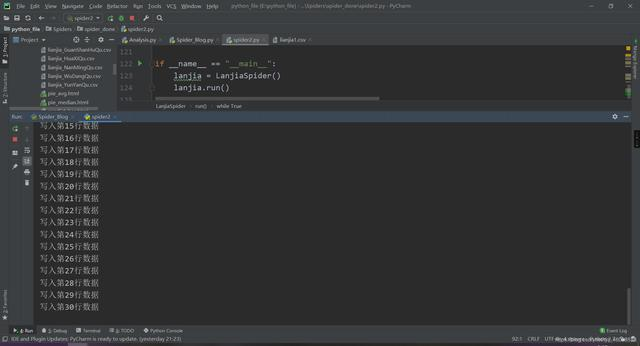然后我们看一下结果：

然后爬虫阶段就结束了，当然也可以写入数据库中，我们保存在文本文件中是为了更方便。我们保存在了左边的csv文件中，是不是很简单~，源码这个网上应该也有，我就暂时不放了，等朋友毕业再发。
二：数据清洗与提取
1:首先导入一下需要的库
"""
数据分析及可视化
"""
import pandas as pd
from pyecharts.charts import Line, Bar
import numpy as np
from pyecharts.globals import ThemeType
from pyecharts.charts import Pie
from pyecharts import options as opts
2：数据全局定义：
places = ['lianjia_BaiYunQu', 'lianjia_GuanShanHuQu', 'lianjia_HuaXiQu', 'lianjia_NanMingQu', 'lianjia_WuDangQu', 'lianjia_YunYanQu']
place = ['白云区', '观山湖区', '花溪区', '南明区', '乌当区', '云岩区']
avgs = []  # 房价均值
median = []  # 房价中位数
favourate_avg = []  # 房价收藏人数均值
favourate_median = []  # 房价收藏人数中位数
houseidfo = ['2室1厅', '3室1厅', '2室2厅', '3室2厅', '其他']  # 房型定义
houseidfos = ['2.1', '3.1', '2.2', '3.2']
sum_house = [0,  0, 0, 0, 0]  # 各房型数量
price = []  # 房价
fav = []  # 收藏人数
type = []
area = []  # 房间面积
注释写的很清楚了，我的places是为了方便读取这几个csv中文件各自保存的数据（‘白云区’, ‘观山湖区’, ‘花溪区’, ‘南明区’, ‘乌当区’, '云岩区’区的数据）：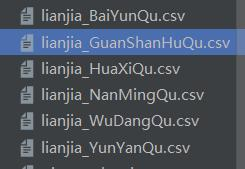3：文件操作，打开文件：
def avg(name):
pattern = '\d+'
df['totalPrice/万元'] = df['totalPrice/万元'].str.findall(pattern) # 转换成字符串，并且查找只含数字的项
df['followInfo'] = df['followInfo'].str.findall(pattern)
df['houseInfo'] = df['houseInfo'].str.findall(pattern)
然后是一些匹配，转换成字符串，并且查找只含数字的项。
for i in range(len(df)):
if (i + 1) % 2 == 0:
continue
else:
if len(df['totalPrice/万元'][i]) == 2:
avg_work_year.append(','.join(df['totalPrice/万元'][i]).replace(',', '.'))
medians.append(float(','.join(df['totalPrice/万元'][i]).replace(',', '.')))
price.append(','.join(df['totalPrice/万元'][i]).replace(',', '.'))
if len(df['followInfo'][i]) ==2:
favourates.append(int(','.join(df['followInfo'][i][:1])))
fav.append(int(','.join(df['followInfo'][i][:1])))
if float(','.join(df['houseInfo'][i][:2]).replace(',', '.')) == 2.1:
k +=1
sum_houses =k
type.append(2.1)
if float(','.join(df['houseInfo'][i][:2]).replace(',', '.')) == 3.1:
k1 +=1
sum_houses =k1
type.append(3.1)
if float(','.join(df['houseInfo'][i][:2]).replace(',', '.')) == 2.2:
k3 +=1
sum_houses =k3
type.append(2.2)
if float(','.join(df['houseInfo'][i][:2]).replace(',', '.')) == 3.2:
k4 +=1
sum_houses =k4
type.append(3.2)
else:
k4 +=1
sum_houses = k4
type.append('other')
area.append(float(','.join(df['houseInfo'][i][2:4]).replace(',', '.')))
sum_house =sum_houses
sum_house = sum_houses
sum_house = sum_houses
sum_house = sum_houses
sum_house = sum_houses

favourates.sort()
favourate_median.append(int(np.median(favourates)))
medians.sort()
median.append(np.median(medians))
# price = avg_work_year
b = len(avg_work_year)
b1= len(favourates)

sum = 0
sum1 = 0
for i in avg_work_year:
sum = sum+float(i)
avgs.append(round(sum/b, 2))
for i in favourates:
sum1 = sum1+float(i)
favourate_avg.append(round(int(sum1/b1), 2))
4：这里是数据筛选的核心部分，我们细说一下：
if len(df['totalPrice/万元'][i]) == 2:
avg_work_year.append(','.join(df['totalPrice/万元'][i]).replace(',', '.'))
medians.append(float(','.join(df['totalPrice/万元'][i]).replace(',', '.')))
price.append(','.join(df['totalPrice/万元'][i]).replace(',', '.'))
5：这里是获取总价格，并且清洗好,放入前面定义好的数组中，保存好，
if len(df['followInfo'][i]) ==2:
favourates.append(int(','.join(df['followInfo'][i][:1])))
fav.append(int(','.join(df['followInfo'][i][:1])))
6：这里是获取总收藏人数，并且清洗好,放入前面定义好的数组中，保存好，
if len(df['followInfo'][i]) ==2:
favourates.append(int(','.join(df['followInfo'][i][:1])))
fav.append(int(','.join(df['followInfo'][i][:1])))
if float(','.join(df['houseInfo'][i][:2]).replace(',', '.')) == 2.1:
k +=1
sum_houses =k
type.append(2.1)
if float(','.join(df['houseInfo'][i][:2]).replace(',', '.')) == 3.1:
k1 +=1
sum_houses =k1
type.append(3.1)
if float(','.join(df['houseInfo'][i][:2]).replace(',', '.')) == 2.2:
k3 +=1
sum_houses =k3
type.append(2.2)
if float(','.join(df['houseInfo'][i][:2]).replace(',', '.')) == 3.2:
k4 +=1
sum_houses =k4
type.append(3.2)
else:
k4 +=1
sum_houses = k4
type.append('other')
area.append(float(','.join(df['houseInfo'][i][2:4]).replace(',', '.')))
7：这里是获取房型和面积，清洗好，放入数组中
favourates.sort()
favourate_median.append(int(np.median(favourates)))
medians.sort()
median.append(np.median(medians))
# price = avg_work_year
b = len(avg_work_year)
b1= len(favourates)

sum = 0
sum1 = 0
for i in avg_work_year:
sum = sum+float(i)
avgs.append(round(sum/b, 2))
for i in favourates:
sum1 = sum1+float(i)
favourate_avg.append(round(int(sum1/b1), 2))
8：这里是把上面的信息加工，生成平均数，中位数等。
另外说一下，清洗过程：’,’.join(）是为了筛选出的信息不含中括号和逗号
df[‘houseInfo’][i][2:4]是为了取出相应的数据，使用了python的切片操作
.replace(’,’, ‘.’)是把逗号改成小数点，这样就是我们想要的结果了。
下面执行看一下结果：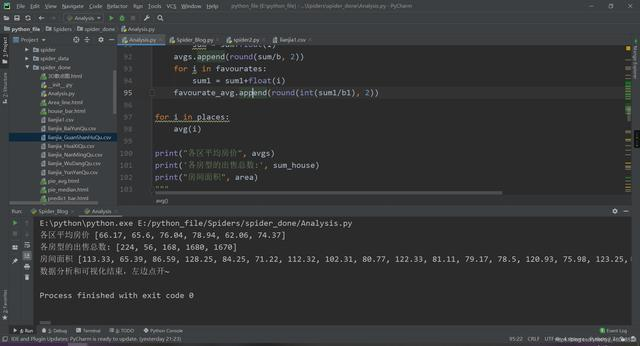数据筛选结束~
由于篇幅过长，贴不出来  需要完整的教程或者源码的加下群：1136192749

展开全文python java 大数据
• ## NER文本数据集标注爬坑

千次阅读 热门讨论 2019-06-19 21:02:47
写在前面：本篇博客记录自己研究在老板的乱七八糟的指挥下踏进了NLP的坑，并且在一段时间调研后发现老板定的方向没有公开数据集（呵呵呵呵~），然后自己苦逼的开始边学技术边搞数据，真是苦不堪言… 一. 数据标注...
•  这里的野数据集指的是从互联网上取出的原始人脸数据集，它的特点是：脏、乱、杂 。数据分布不均匀、尺寸不一、存在年龄跨度较大现象，图像格式命名错误且存在较多的冗余、杂质，但优点是类别数目大。使用前需要...
• 这里涉及到的数据集是京东最新的数据集： JData_User.csv 用户数据集 105,321个用户 JData_Comment.csv 商品评论 558,552条记录 JData_Product.csv 预测商品集合 24,187条记录 JData_Action_201602.csv 2月份行为...算法 python 大数据 机器学习
• python-matplotlib 一、matplotlib绘图要点： plt.figure(1) ## 初始化一张画布，可以自定义图像 plt.subplot(211) #创建单个子图，subplots创建多个子图 plt.plot(A,B) #直接在一张大的画布中画图 ...
• 主要是基于豆瓣电影的数据，进行分析，所以首先要爬取相关的电影数据，对应的源代码在DouBan_Spider目录下，主要是采用Python + BeautifulSoup + urllib进行数据采集 2:ETL预处理 3:数据分析 4:可视化 代码封装...
• 购买变量的数据清洗 ‘人付款’需要去掉 7.5万+需要转换为75000 9500+需要去掉+ 解题思路 ‘人付款’可以用空格替换掉 用正则表达式取出数字 数字处理用if语句判断数据单位是否带‘万’处理 代码 #载入各种包 ...python
•爬虫 xml 情感分析
• 明确目标-》采集数据-》数据清洗与分析-》绘制图表并且可视化-》得出结论 1.2 具有python特色的程序 1.3 字符串切片 1 循环打印嵌套列表：movies=[“the holy”,1975,“terry jones”,91,[“graham”, [“michael”...爬虫 python
• 由武汉大学多媒体研究中心发起，目前是全球最大的口罩遮挡人脸数据集。分为真实口罩人脸和模拟口罩人脸两部分，真实口罩人脸包括525人的5000张口罩人脸和9万张正常人脸。模拟口罩人脸包含1万个人共50万张模拟人脸...目标识别
• 图片到图片装载器、绘制火焰效果的X坐标,Y坐标、得到X坐标,Y坐标值、绘制火焰效果Image…… Java加密解密工具 JCT v1.0源码包 5个目标文件 内容索引:JAVA源码,综合应用,JCT,加密解密 WDSsoft的一款免费源代码 JCT ...java
• ## ETL数据清洗概述

千次阅读 2020-06-30 15:44:47
数据清洗（Data Cleaning）原理即通过分析“脏数据”的产生原因和存在形式，利用现有的技术手段和方法去清洗“脏数据”,将原有的不符合要求的数据转化为满足数据质量或应用要求的数据，从而提高数据集的数据质量。...
• 表示任何单个字符 [] 字符，对单个字符给出取值范围 [abc]表示a,b,c [^] 非字符，对单个字符给出排除范围 [^abc]表示非a或b或c的单个字符 * 前一个字符0次或无限次扩展 abc*表示ab,abc,abcc等 + 前一个字符1次...爬虫 python
• 实例将 EMPLOYEE 表中的 SEX 字段为 ‘M’ 的 AGE 字段递增 1： # SQL 更新语句 sql = "UPDATE EMPLOYEE SET AGE = AGE + 1 WHERE SEX = '%c'" % ('M') 七、删除操作 删除操作用于删除数据表中的数据，以下实例演示...python mysql
• 目录1....2.正则表达式做数据清洗 2.1 从网页HTML标签中提取文本 import re text = "<div><p>\n你好\nPython：</p><p>Python是一种跨平台的计算机程序设计语言。 </p><python 正则表达式
• 1、数据清洗的方法 1、缺失值处理：对存在缺失的数据进行插补 2、异常值处理：对数据集中存在的不合理值进行处理 3、数据转换：将数据从一种表现形式转换成另一种表现形式 2、数据清洗的工具 1、Mapreduce(Hadoop)...python 爬虫
• 《Python3爬虫、数据清洗和可视化实战》 零一 韩要宾 黄园园 著 第五章 Scrapy爬虫 5.1 Scrapy简介 Scrapy是为了抓取网页数据、提取结构化数据而编写的应用框架。该框架是封装的，包含request、下载器、解析器、...
•python nlp 正则表达式
• 数据挖掘】数据清洗数据挖掘一般流程 数据挖掘一般流程机器学习
• 数据集来源于某个比赛，文件是.json格式，内部嵌套了多个字典： json文件中的每一项的键是论文id,值是一个字典，该字典又包含键authors(作者)、title(论文题目)、abstract(摘要)、keywords(关键字)、venue(期刊)、...
• 房天下网站二手房爬虫、数据清洗及可视化（python） 爬虫代码 ###爬取完的数据存入MangoDB中，需自行下载MangoDB import requests, json, threading from bs4 import BeautifulSoup import numpy as np import re ...python
• 阿里巴巴的数据相对来说更为简单，数据字段信息较为简单，也是选用阿里巴巴的数据集作为分析案例的主要原因。初步看来，所有的电商数据集都会有用户，商品，以及时间字段。而不同的字段进行组合可以带来其他有用信息...
• 前言 如果你有什么问题，希望跟我能够一起交流，除了通过博客交流外，欢迎你加入我的...YOLO有自己训练好的数据集，在YOLO v2 中，数据集可检测的类别达9000种以上，但是9000毕竟不是全部，它能涵盖大部分的物体识...LabelIMg...